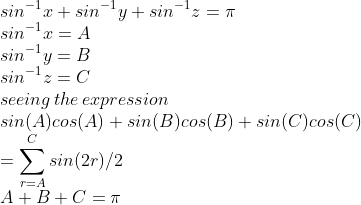# if sin -1x +sin-1 y+ sin-1 z= pie ,prove thata)x( root 1-x2)+y( root 1- y2)+ z(root 1-z2)= 2xyz

9 years ago

Let sin-1x =A then x= sinA

Let sin-1y=B then y =sinB

Let sin-1z=C then z=sinC

Then A+B+C =p

Xv(1-X2) +Yv(1-Y2) +Zv(1-Z2)=sinA v(1-sin2A)+sinBv(1-sin2B) +sinCv(1-sin2C)

=sinA.cosA+sinB.cosB+sinC.cosC

=1/2[sin2A+sin2B+sin2C]

=1/2 [4sinA.sinB.sinC]

=2sinAsinBsinC

=2xyz

Thanks & Regards

Rinkoo GuptaArun Kumar IIT Delhi
9 years ago
Hi Ashu,

Thanks & Regards, Arun Kumar, Btech,IIT Delhi, Askiitians FacultyArun Kumar IIT Delhi
9 years ago
AshuArun Kumar IIT Delhi
9 years ago
Hi Ashu,
So required expression will become

Thanks & Regards, Arun Kumar, Btech,IIT Delhi, Askiitians Faculty

3 years ago
hello studentHope it helps
Thannkyou